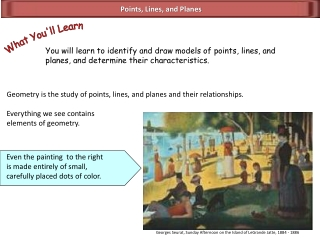DownloadDownload PresentationPoints, Lines, and Planes

# Points, Lines, and Planes

Télécharger la présentation## Points, Lines, and Planes

- - - - - - - - - - - - - - - - - - - - - - - - - - - E N D - - - - - - - - - - - - - - - - - - - - - - - - - - -
##### Presentation Transcript

1. Even the painting to the right is made entirely of small, carefully placed dots of color. Georges Seurat, Sunday Afternoon on the Island of LeGrande Jatte, 1884 - 1886 Points, Lines, and Planes What You'll Learn You will learn to identify and draw models of points, lines, andplanes, and determine their characteristics. Geometry is the study of points, lines, and planes and their relationships.Everything we see contains elements of geometry.

2. A B Points, Lines, and Planes point A ____ is the basic unit of geometry. POINT: A point has no ____. size Points are named using capital letters. The points at the right are named point A and point B.

3. A B The symbol for line AB is l Points, Lines, and Planes A ____is a series of points that extends without end in two directions. line infinite number LINE: A line is made up of an ______ _______ of points. arrows The ______ show that the line extends without end in both directions. A line can be named with a single lowercase script letter or by two points on the line. The line below is named line AB, line BA, or line l.

4. m R T S or line m Points, Lines, and Planes 1) Name two points on line m. Possible answers: point R and point S point R and point T point S and point T 2) Give three names for the line. Possible answers: NOTE: Any two points on the line or the script letter can be used to name it.

5. R T V S U 1) Name three points that are collinear. Possible answers: Points, Lines, and Planes collinear Three points may lie on the same line. These points are _______ . noncollinear Points that DO NOT lie on the same line are __________ . points R, S, and point T points U, S, and point V

6. R T V S U 1) Name three points that are noncollinear. Possible answers: Points, Lines, and Planes collinear Three points may lie on the same line. These points are _______ . noncollinear Points that DO NOT lie on the same line are __________ . points R, T, and point U points R, S, and point V points R, S, and point U points R, T, and point V points R, V, and point U points S, T, and point V

7. B A RAY: The starting point of a ray is called the ________. The symbol for ray AB is Points, Lines, and Planes Rays and line segments are parts of lines. ray A ___ has a definite starting point and extends without end in one direction. endpoint A ray is named using the endpoint first, then another point on the ray. The ray above is named ray AB.

8. B A The symbol for segment AB is Points, Lines, and Planes Rays and line segments are parts of lines. line segment A ___________ has a definite beginning and end. LINE SEGMENT: A line segment is part of a line containing two endpoints and all points between them. A line segment is named using its endpoints. The line segment above is named segment AB or segment BA.

9. Points, Lines, and Planes plane A _____ is a flat surface that extends without end in all directions. coplanar Points that lie in the same plane are ________. noncoplanar Points that do not lie in the same plane are ___________. PLANE: For any three noncollinear points, there is only one plane that contains all three points. A M A plane can be named with a single uppercase script letter or by three noncollinear points. B C The plane at the right is named plane ABC or plane M.

10. C A B E D Hands On Place points A, B, C, D, & E on a piece of paper as shown. Fold the paper so that point A is on the crease. Open the paper slightly. The two sections of the paper represent different planes. Answers (may be others) A, B, & C 1) Name three points that are coplanar. ______________________ D, A, & B 2) Name three points that are noncoplanar. ______________________ A 3) Name a point that is in both planes. ______________________